• pythonnumpy
千次阅读
2021-12-09 16:00:11

## 生成随机数 ,NumPy提供了random模块来处理随机数。

### 1.生成一个从0到100的随机整数：

from numpy import random

x = random.randint(100)

print(x)

### 2.生成随机浮点数(float)

随机模块的rand()方法返回0到1之间的随机浮点数。

例如：

生成从0到1的随机浮点数：

from numpy import random

x = random.rand()

print(x)

### 3、生成随机数组

在NumPy中，使用数组，可以使用上面示例中的两种方法来创建随机数组。

Integers

randint()方法采用一个size参数，可以在其中指定数组的形状。

例如：

生成一维数组，其中包含5个从0到100的随机整数：

from numpy import random

x=random.randint(100, size=(5))

print(x)

例如：

生成具有3行的2-D数组，每行包含5个从0到100的随机整数：

from numpy import random

x = random.randint(100, size=(3, 5))

print(x)

Floats

rand()方法还允许您指定数组的形状。

例如：

生成一个包含5个随机浮点数(float)的1-D数组:

from numpy import random

x = random.rand(5)

print(x)

例如：

生成具有3行的二维数组，每行包含5个随机数：

from numpy import random

x = random.rand(3, 5)

print(x)

### 4.从数组中生成随机数

choice()方法允许您基于值数组生成随机值。

choice()方法将数组作为参数，并随机返回其中一个值。

例如：

返回数组中的值之一：

from numpy import random

x = random.choice([3, 5, 7, 9])

print(x)

choice()方法还允许您返回值的array

添加size参数以指定数组的形状。

例如：

生成一个二维数组，由数组参数(3,5,7,9)中的值组成:

from numpy import random

x = random.choice([3, 5, 7, 9], size=(3, 5))

print(x)python
更多相关内容
• ## PythonNumpy矩阵乘法

千次阅读 2020-09-15 18:41:23
In this tutorial we will see python matrix multiplication using numpy (Numerical Python) library. 在本教程中，我们将看到使用numpy(Numerical Python)库的python矩阵乘法 。 For using numpy you must ...

In this tutorial we will see python matrix multiplication using numpy (Numerical Python) library.

在本教程中，我们将看到使用numpy(Numerical Python)库的python矩阵乘法

For using numpy you must install it first on your computer, you can use package manager like pip for installing numpy.

要使用numpy，必须先将其安装在计算机上，然后可以使用软件包管理器(如pip)安装numpy。

Numpy provide array data structure which is almost the same as python list but have faster access for reading and writing resulting in better performance. We will use numpy arrays to represent matrices.

Numpy提供的数组数据结构与python列表几乎相同，但具有更快的读写访问权限，从而提高了性能。 我们将使用numpy数组来表示矩阵。

To perform matrix multiplication of matrices a and b , the number of columns in a must be equal to the number of rows in b otherwise we cannot perform matrix multiplication.

为了执行矩阵AB的矩阵乘法， 列数必须等于b中的行否则就不能执行矩阵乘法的数量。

We must check this condition otherwise we will face runtime error.

我们必须检查这种情况，否则我们将面临运行时错误。

There is * operator for numpy arrays but that operator will not do matrix multiplication instead it will multiply the matrices element by element.

numpy数组有*运算符，但该运算符不会进行矩阵乘法，而是将矩阵元素逐元素相乘。

Here is an example with * operator:

这是带有*运算符的示例：

# Import numpy
import numpy as np

def printMatrix(a):

# Printing matrix
for i in range(0,len(a)):
for j in range(0,len(a)):
print(a[i][j],end = " ")
print()

def main():

# Declaring our matrices using arrays in numpy
a = np.array([[1,2,3],[3,4,5],[5,6,7]])
b = np.array([[1,2,3]])

print("Matrix a :")
printMatrix(a)
print()

print("Matrix b : ")
printMatrix(b)
print()

# Using * operator to multiply
c = a*b

# Printing Result
print("Result of a*b : ")
printMatrix(c)

main()

Output:

输出：

Matrix a : 1 2 3 3 4 5 5 6 7

矩阵a： 1 2 3 3 4 5 5 6 7

Matrix b : 1 2 3

矩阵b： 1 2 3

Result of a*b : 1 4 9 3 8 15 5 12 21

a * b的结果： 1 4 9 3 8 15 5 12 21

## Python Numpy矩阵乘法 (Python Numpy Matrix Multiplication)

We can see in above program the matrices are multiplied element by element. So for doing a matrix multiplication we will be using the dot function in numpy.

我们可以在上面的程序中看到矩阵乘以一个元素。 因此，为了进行矩阵乘法，我们将在numpy中使用点函数。

We can either write

我们可以写

• np.dot(a,b)

np.dot(a，b)
• a.dot(b)

点(b)

for matrix multiplication here is the code:

对于矩阵乘法，下面是代码：

# Import numpy
import numpy as np

def printMatrix(a):

# Printing matrix
for i in range(0,len(a)):
for j in range(0,len(a)):
print(a[i][j],end = " ")
print()

def main():

# Taking rows and columns of a
m = int(input("Enter rows in a : "))
n = int(input("Enter columns in a : "))

# Taking rows and columns of b
p = int(input("Enter rows in b : "))
q = int(input("Enter columns in b : "))

# Checking necessary condition for matrix multiplication
if n!= p:
print("Number of columns in b must be equal to rows in b")
exit()

# Initializing a and b list
a = [ [0 for i in range(0,n)] for j in range(0,m) ]
b = [ [0 for i in range(0,q)] for j in range(0,p) ]

# Taking input list a
print("Enter matrix a : ")
for i in range(0,m):
for j in range(0,n):
a[i][j] = int(input("Enter element a[" + str(i) + "][" + str(j) + "] : "))

# Taking input list b
print("Enter matrix b : ")
for i in range(0,p):
for j in range(0,q):
b[i][j] = int(input("Enter element b[" + str(i) + "][" + str(j) + "] : "))

# Converting python list in numpy array
a = np.array(a)
b = np.array(b)

print("Matrix a :")
printMatrix(a)
print()

print("Matrix b : ")
printMatrix(b)
print()

# Using dot operator to multiply
c = a.dot(b)

# Printing Result
print("Result of a*b : ")
printMatrix(c)

main()

Output:

输出：

Enter rows in a : 2 Enter columns in a : 3 Enter rows in b : 3 Enter columns in b : 2 Enter matrix a : Enter element a : 2 Enter element a : 3 Enter element a : 4 Enter element a : 1 Enter element a : 2 Enter element a : 3 Enter matrix b : Enter element b : 4 Enter element b : 5 Enter element b : 1 Enter element b : 6 Enter element b : 9 Enter element b : 7 Matrix a : 2 3 4 1 2 3

输入a中的行：2 输入a中的列：3 输入b中的行：3 输入b中的列：2 输入矩阵a： 输入元素a  ：2 输入元素a  ：3 输入元素a  ：4 输入元素a  ：1 输入元素a  ：2 输入元素a  ：3 输入矩阵b： 输入元素b  ：4 输入元素b  ：5 输入元素b  ：1 输入元素b  ：6 输入元素b  [0 ]：9 输入元素b  ：7 矩阵a： 2 3 4 1 2 3

Matrix b : 4 5 1 6 9 7

矩阵b： 4 5 1 6 9 7

Result of a*b : 47 56 33 38

a * b的结果： 47 56 33 38

Here the output is different because of the dot operator. Alternatively we can use the numpy matrices method to first convert the arrays into matrices and then use * operator to do matrix multiplication as below:

由于点运算符，此处的输出是不同的。 或者，我们可以使用numpy矩阵方法首先将数组转换为矩阵，然后使用*运算符进行矩阵乘法，如下所示：

# Using * operator to multiply
c = np.matrix(a)*np.matrix(b)

Comment below if you have any queries related to python numpy matrix multiplication.

如果您有任何有关python numpy矩阵乘法的查询，请在下面评论。

展开全文数据结构 python 机器学习
• ## pythonnumpy 乘法总结

千次阅读 2021-10-24 23:06:51
import numpy as np a = np.array([[1,2,2],[2,1,2],[3,2,1]]) b = np.array([[1,1,1],[0,1,1],[1,1,0]]) print(a) print(b) #数学上的矩阵乘法 print(a@b) print(np.matmul(a,b)) print(np.dot(a,b)) #行...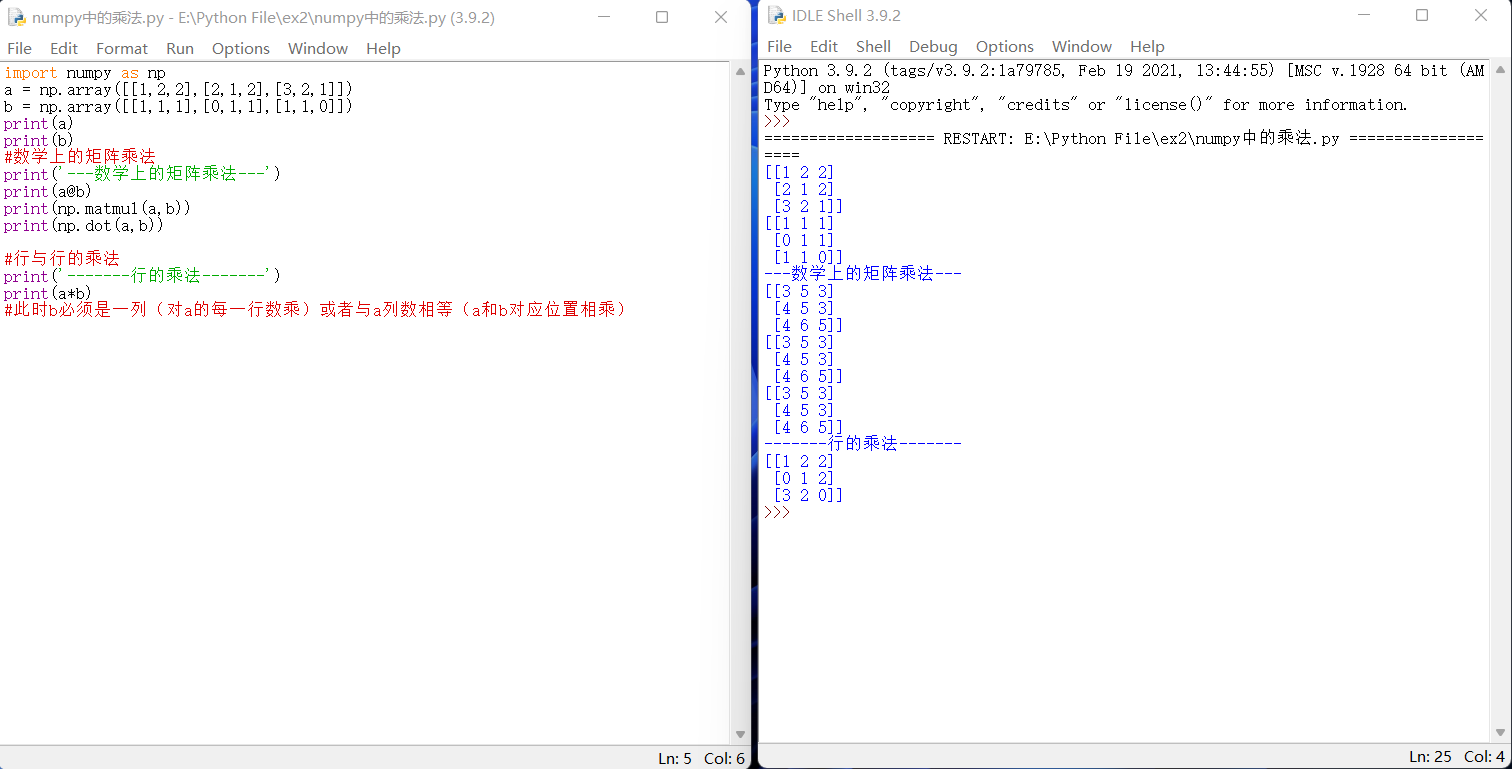​​​​​​​

import numpy as np

a = np.array([[1,2,2],[2,1,2],[3,2,1]])

b = np.array([[1,1,1],[0,1,1],[1,1,0]])

print(a)

print(b)

#数学上的矩阵乘法

print(a@b)

print(np.matmul(a,b))

print(np.dot(a,b))

#行与行的乘法

print(a*b)

#此时b必须是一列（对a的每一行数乘）或者与a列数相等（a和b对应位置相乘）

展开全文python
• ## pythonnumpy库

千次阅读 多人点赞 2021-12-18 20:15:54
NumPy( Numeric Python) numpypython中一个可以用来处理多维数组的库。

## NumPy( Numeric Python)

numpy是一个开源的python科学计算扩展库，主要用来处理任意维度数组和矩阵
相同的任务，使用numpy比直接用python的基本数据结构更加简单高效。
它的功能：

1. 包含一个强大的N维数组对象Ndarray
2. 广播功能函数
3. 整合C/C++代码的工具
4. 线性代数、傅里叶变换、随机数生成等功能
numpy是scipy,pandas等数据处理或科学计算库的基础

## numpy的引用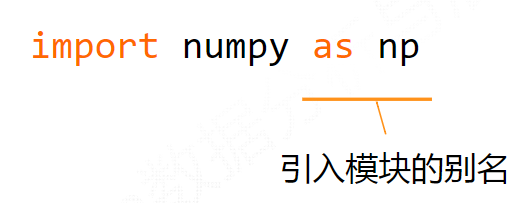虽说别名可以省略或者更改，但尽量使用上述约定的别名

## Ndarray

n维数组，它是一个相同数据类型的集合，以0为下标开始进行集合中元素的索引。
我们知道，python有列表和数组此类的数据结构。
列表：数据类型可以不同（如[3, 2.4 ,‘a’ ,“abc”]），数据是有序的
数组：数据类型相同(如[1，2，3，4])
集合：（如{2，4，3，5，7}）数据是无序的

### 引入n维数组的意义

观察下列两组操作，其功能都是一样的。

import numpy as np

def pysum():
a = [1, 2, 3, 4]
b = [5, 6, 7, 8]
c = []
for i in range(len(a)):
c.append(a[i]**2+b[i]**3)
return c

def numpysum():
a = np.array([1, 2, 3, 4])
b = np.array([5, 6, 7, 8])
c = a**2+b**3
return c

print("使用列表运算的结果是：", pysum())
print("使用Numpy运算的结果是：", numpysum())
'''
运行结果：
使用列表运算的结果是： [126, 220, 352, 528]
使用Numpy运算的结果是： [126 220 352 528]
'''


但是很明显：

1. numpy的数组对象可以去掉元素建运算所需要的循环，使一维向量更像单个数据
2. numpy通过设立专门的数组对象，经过优化，运算速度也相应提升
通常情况下，在科学运算中，一个维度所有数据的类型往往相同，这时，使用数组对象采用相同的数据类型，有助于节省运算时间和存储空间

### ndarray的组成

1. 实际的数据
2. 描述这些数据的元数据（数据维度、数据类型等）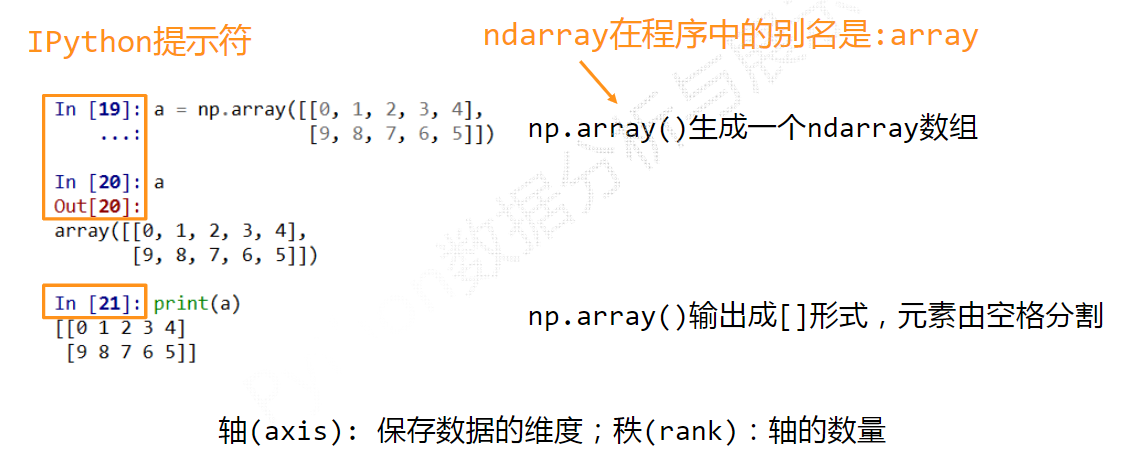### ndarray对象的属性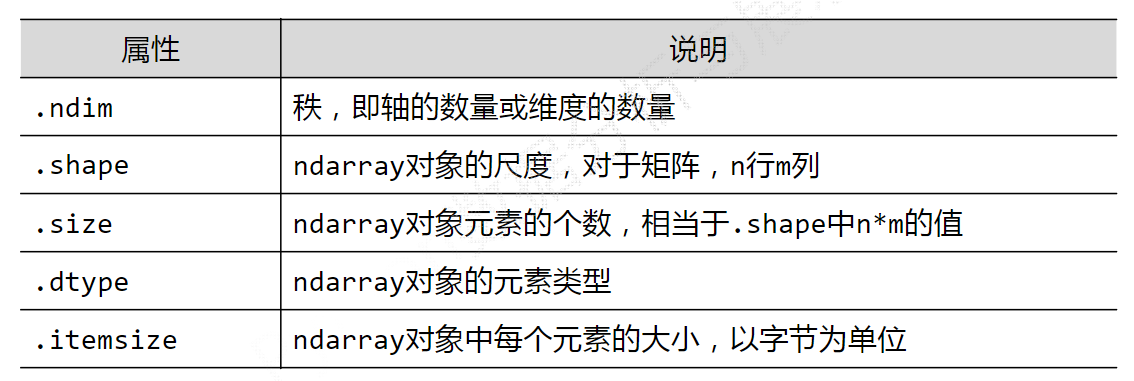## 数据类型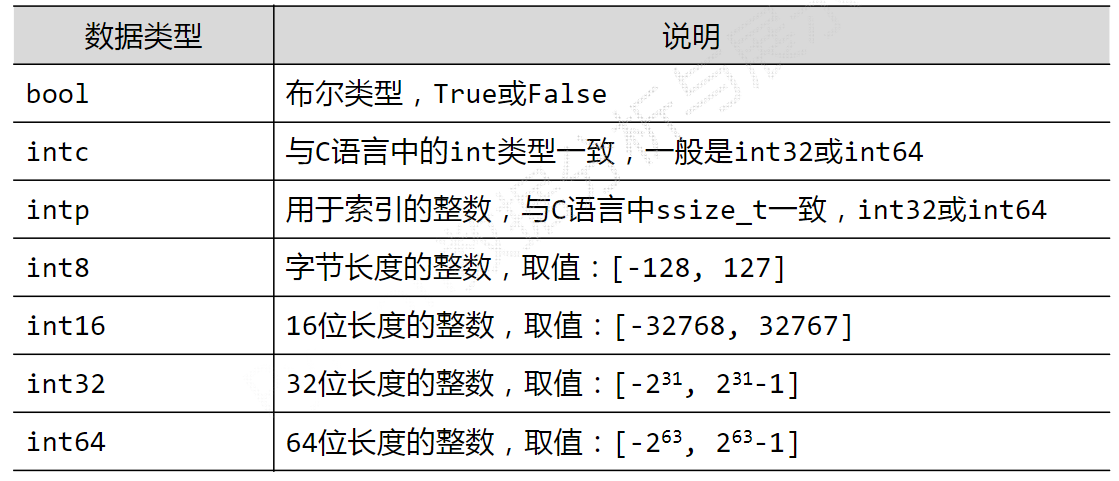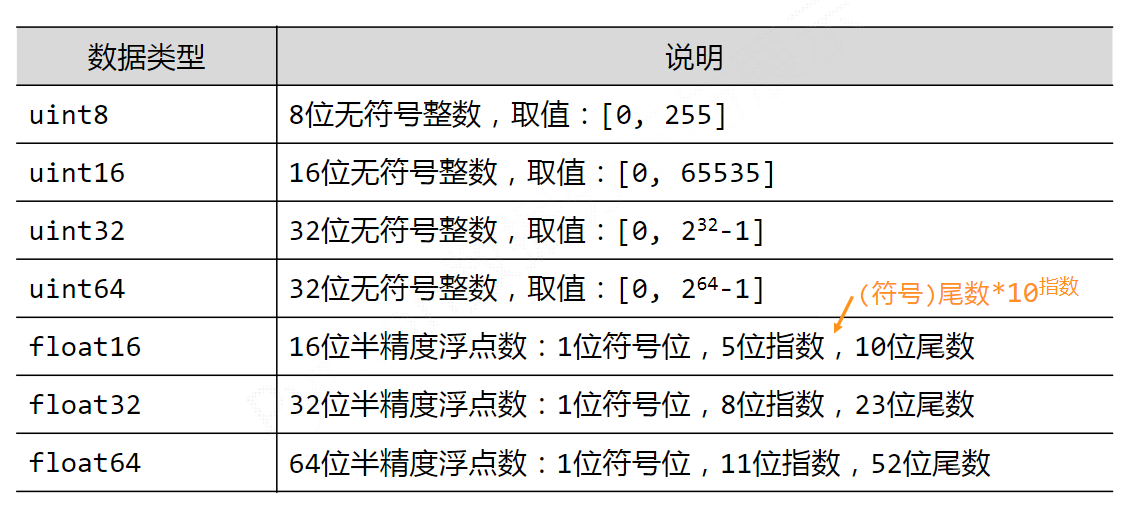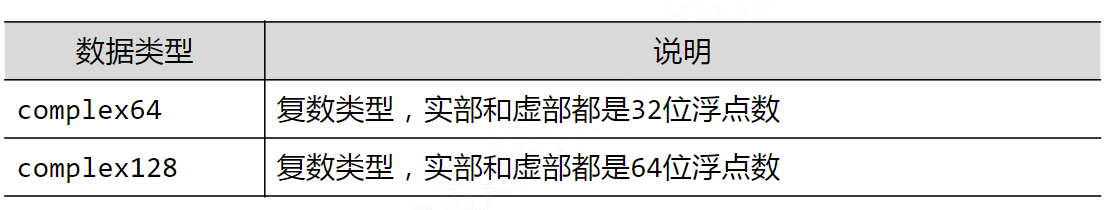当np.array()不指定dtype时，numpy将根据数据情况关联一个dtype类型

### ndarray支持多种数据类型的原因

1. python基本语法只支持整数、浮点数和复数3种类型
2. 科学计算涉及数据较多，对存储和性能都有较高要求
3. 对元素类型精细定义，有助于numpy合理使用存储空间并优化性能
4. 对元素类型精细定义，有助于程序员对程序规模有合理评估

### ndarray数组的创建

import numpy as np
x = np.array([[1, 0], [2, 0], [3, 1]], np.int32)
print(x)
print(x.dtype)
'''
程序输出：
[[1 0]
[2 0]
[3 1]]
int32
'''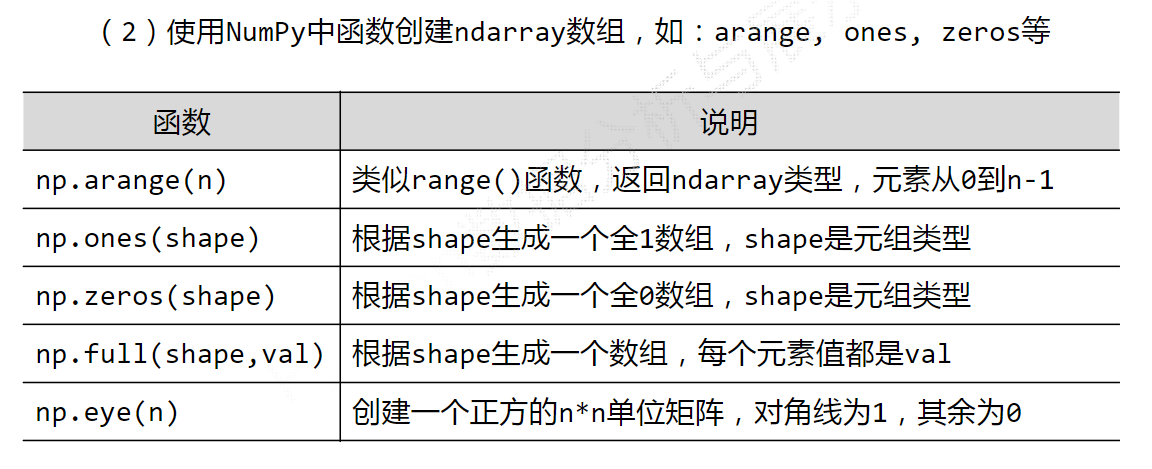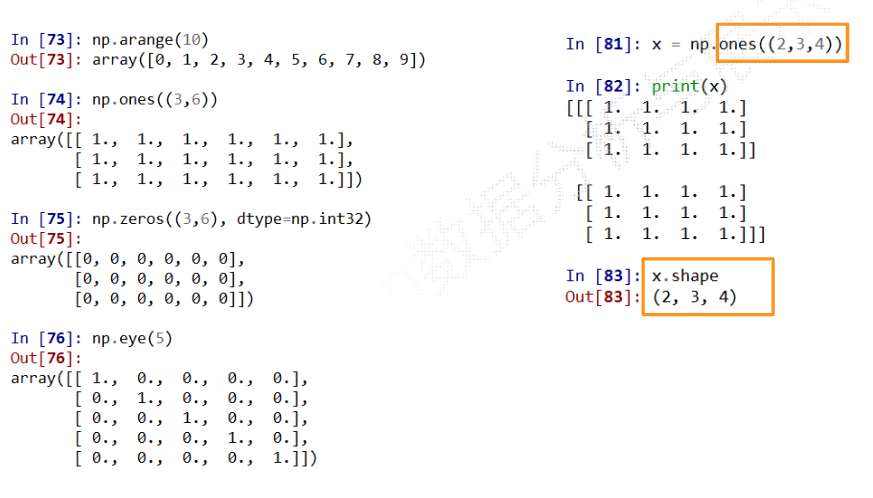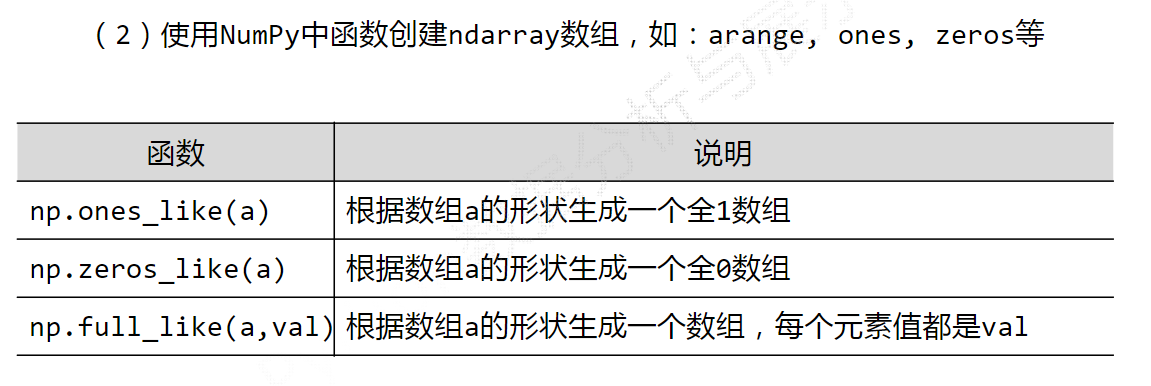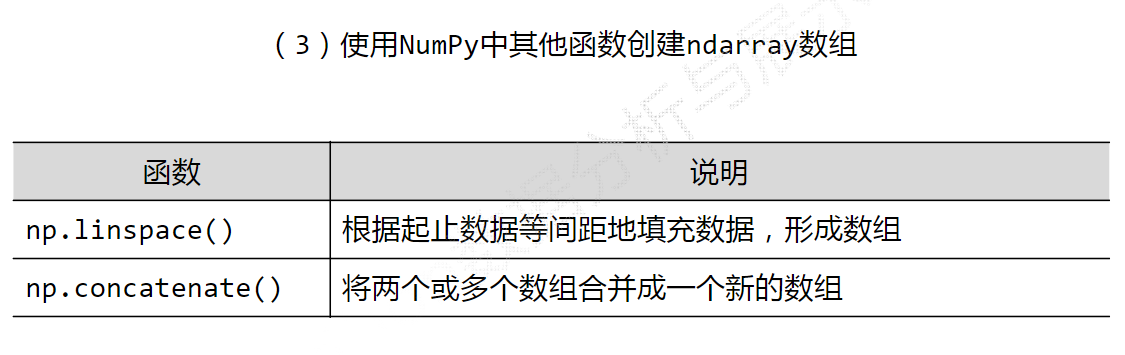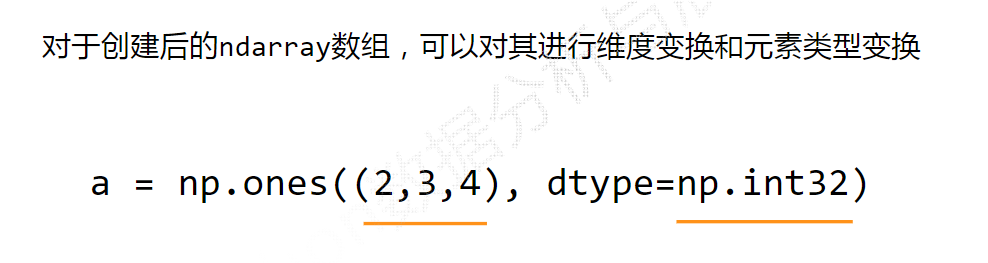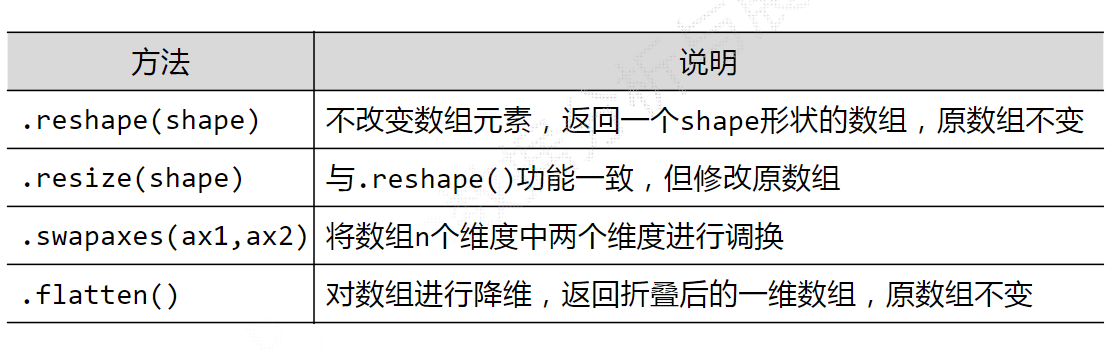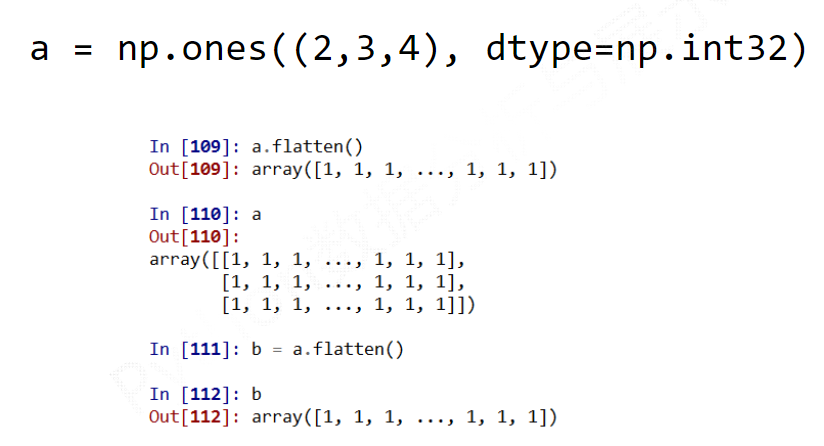### ndarray数组运算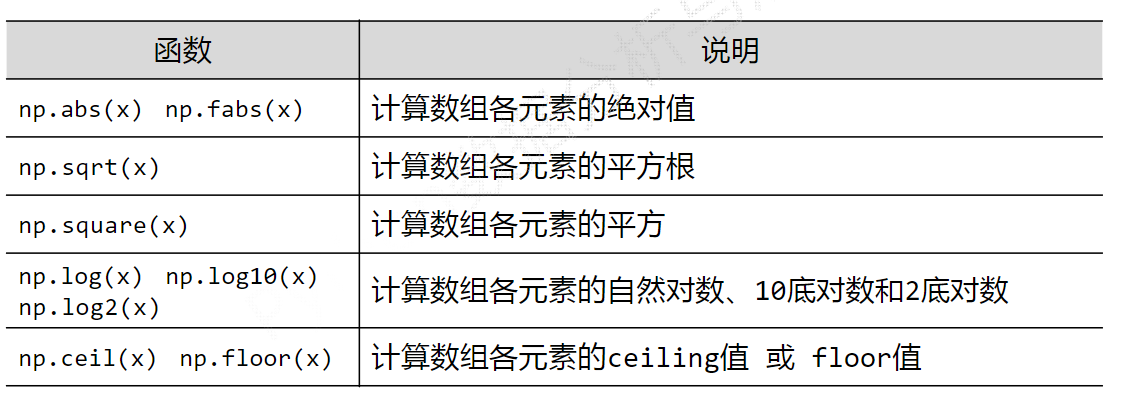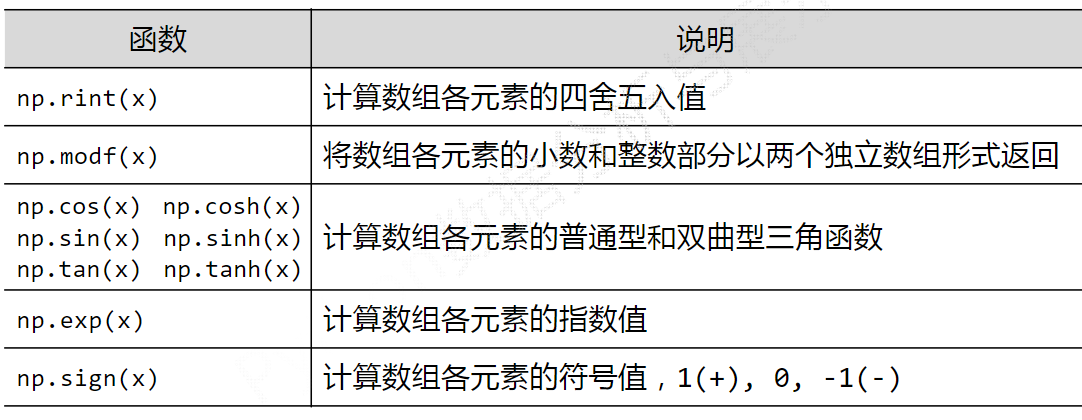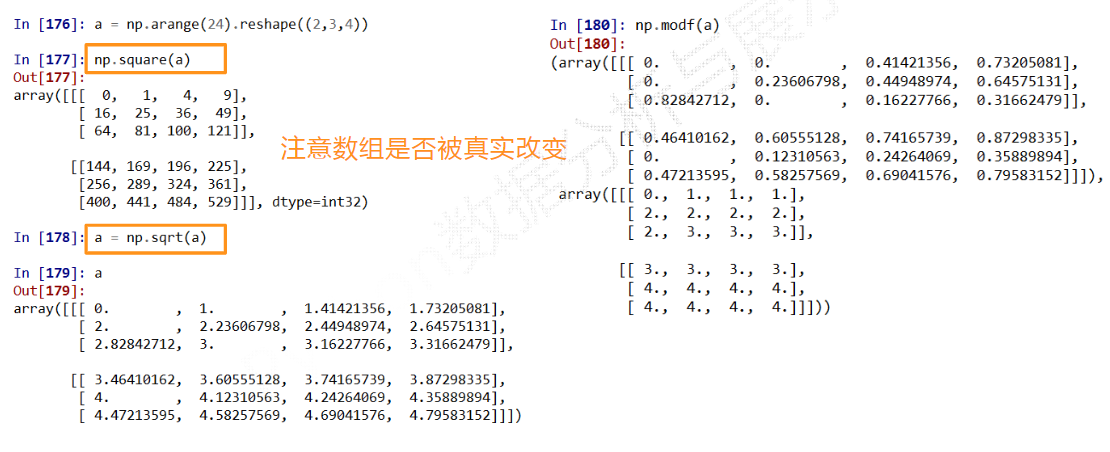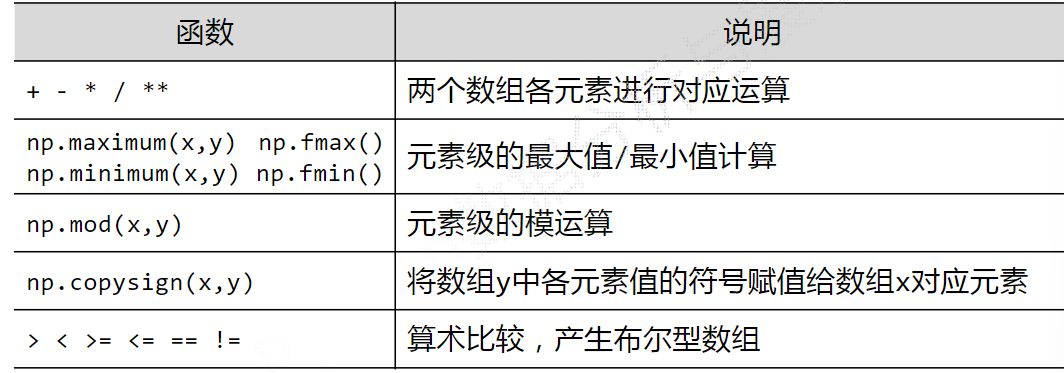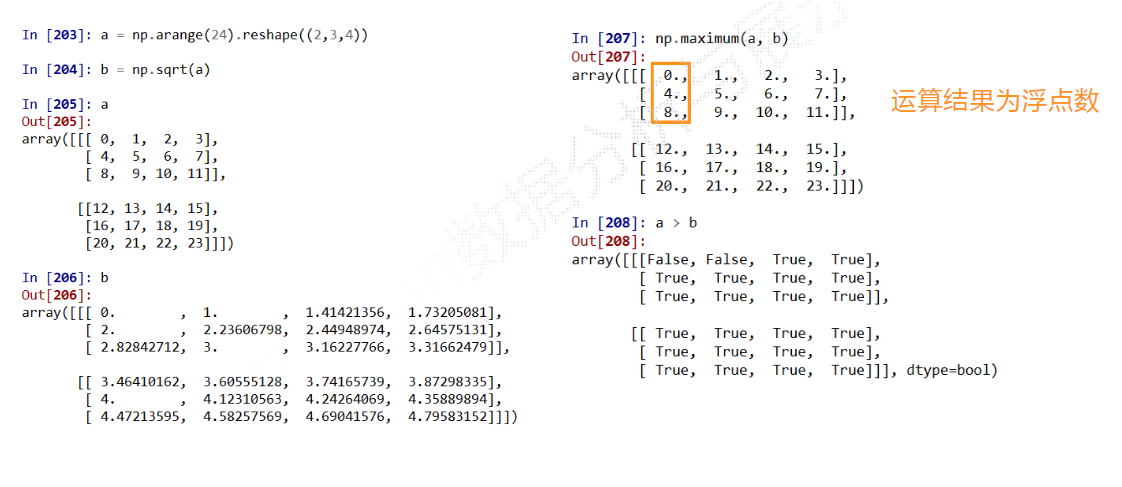## 索引与切片

索引：获取数组中特定位置元素的过程
切片：获取数组元素子集的过程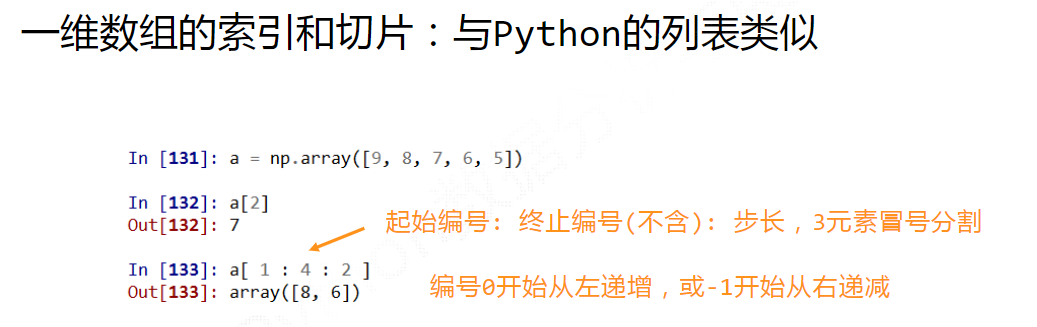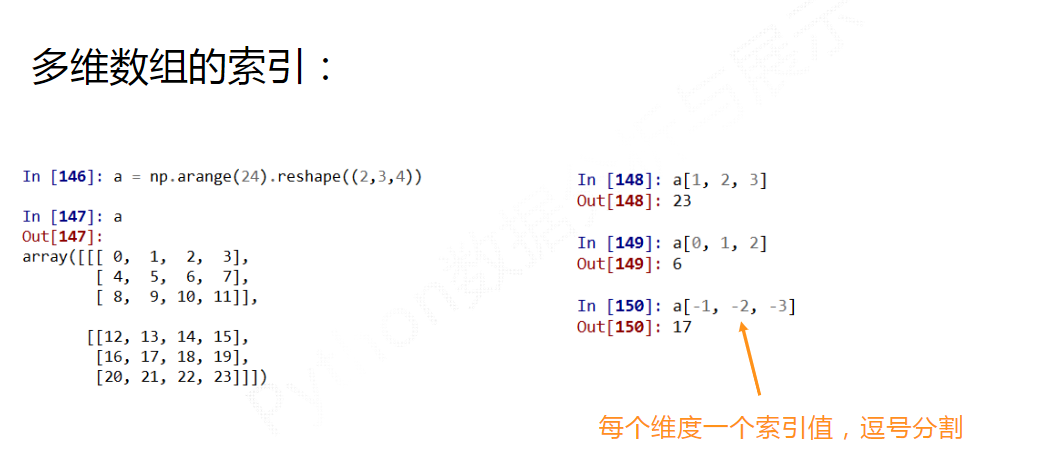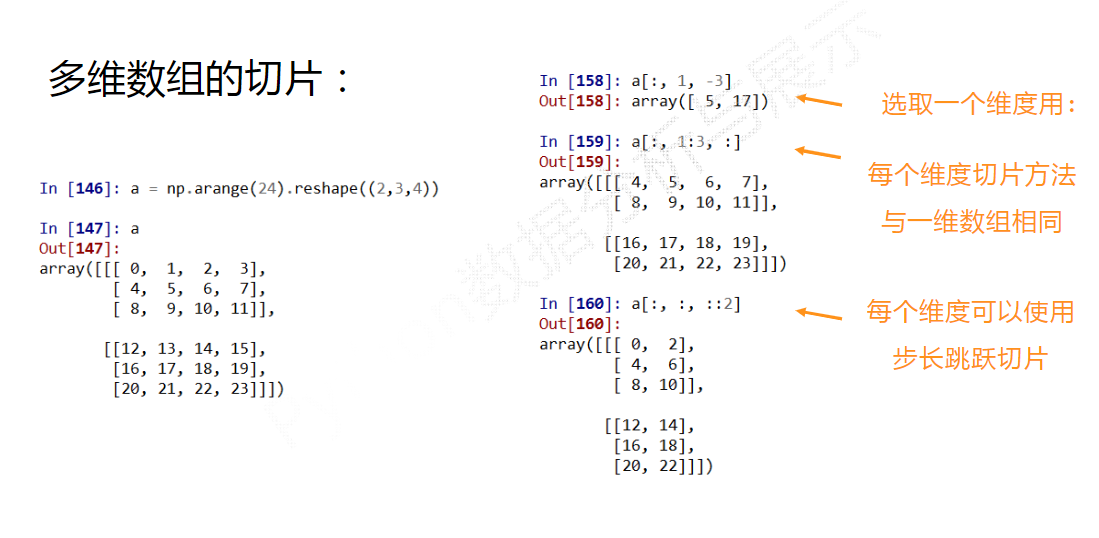## 随机数函数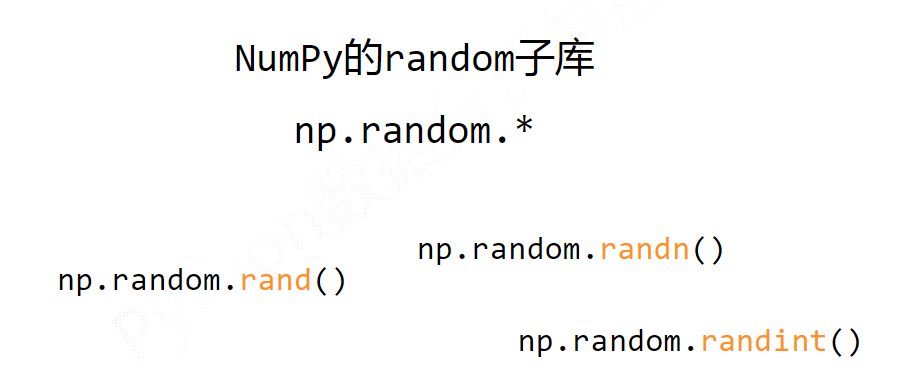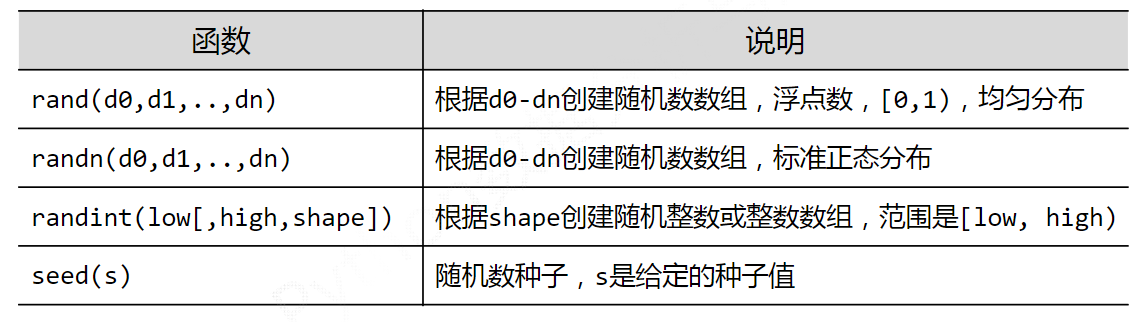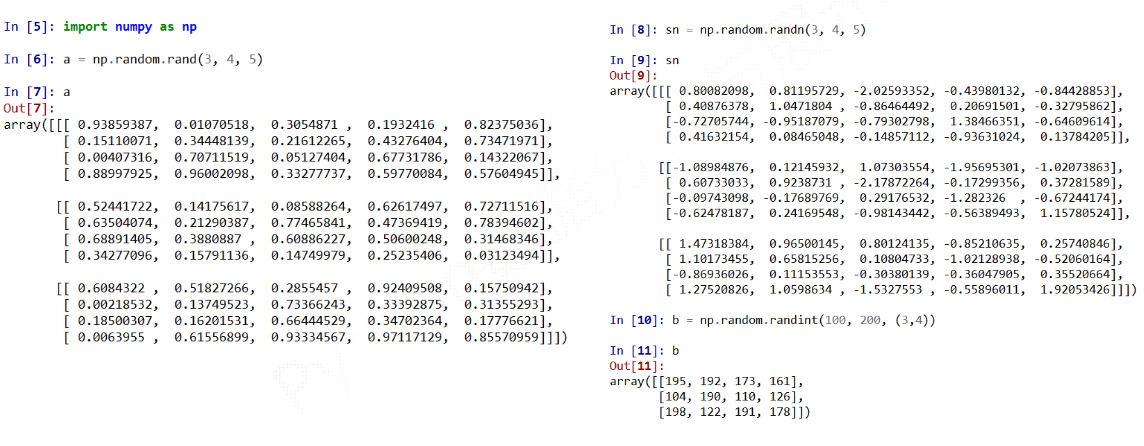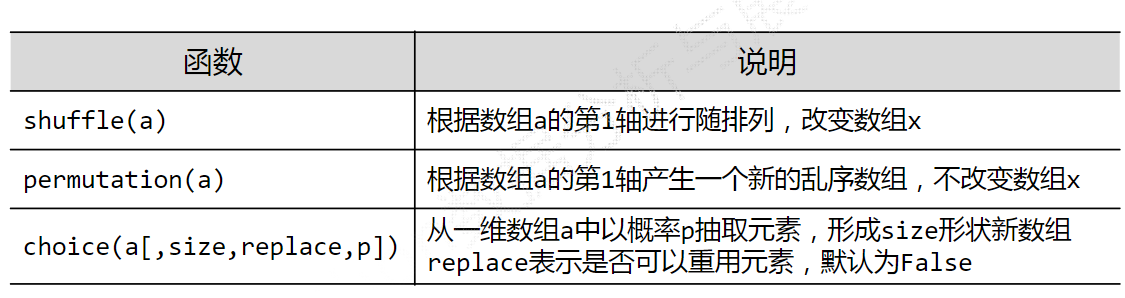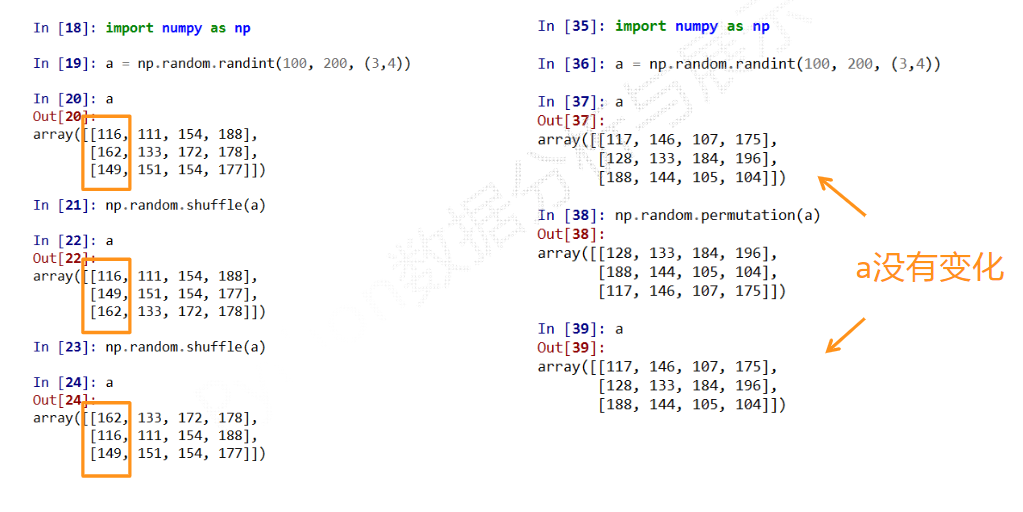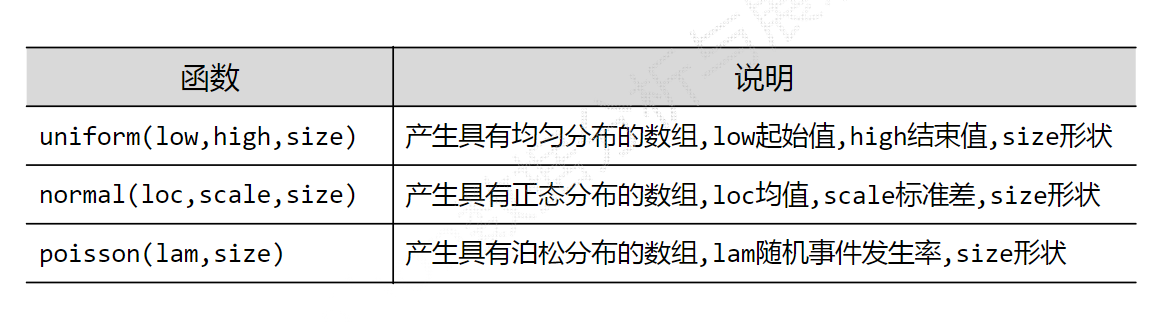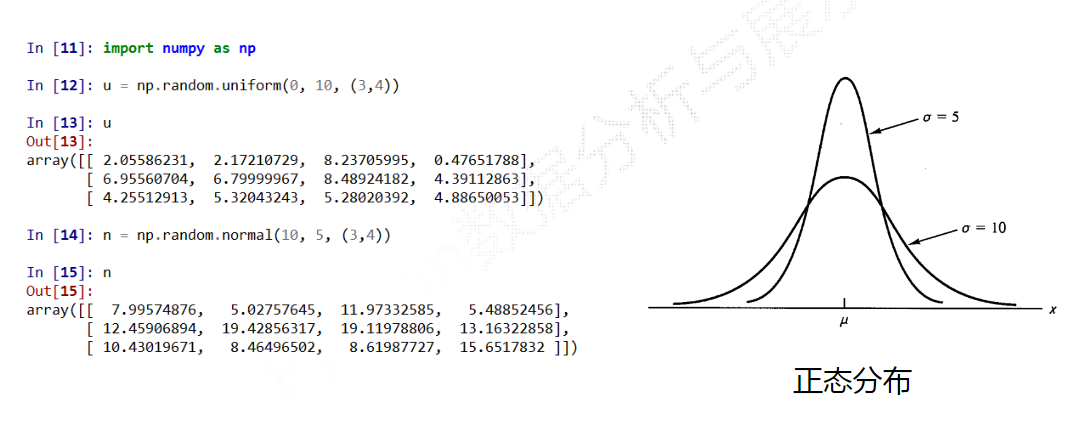## 统计函数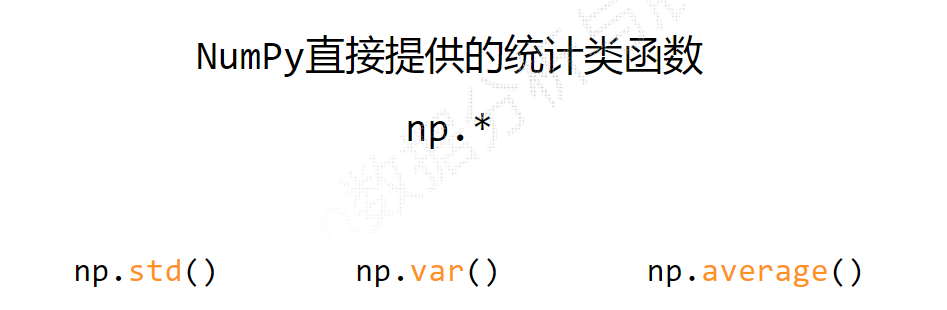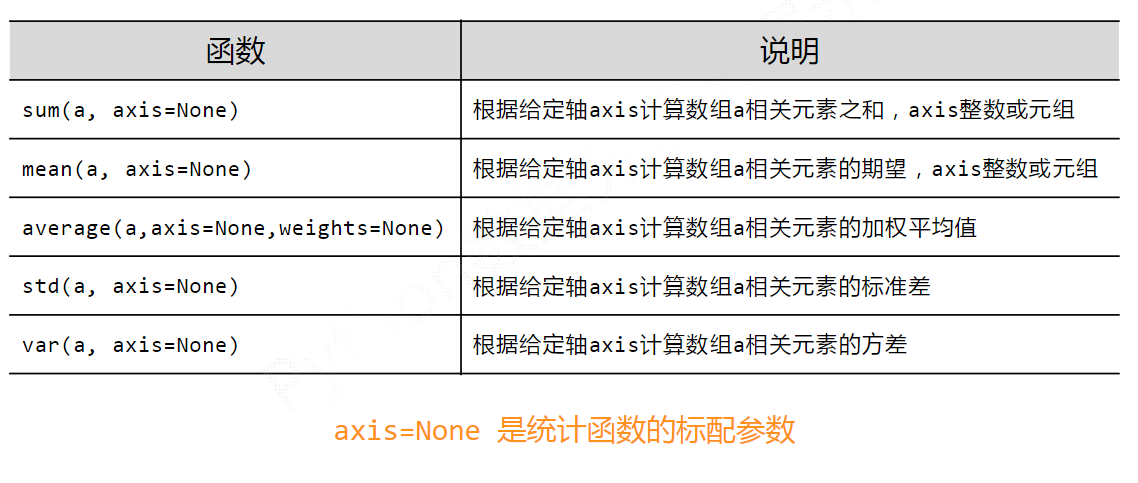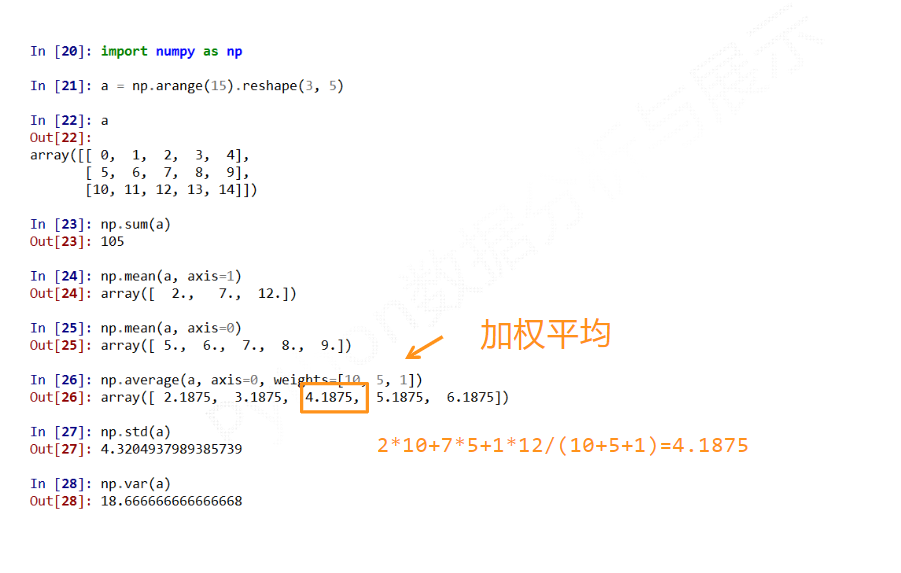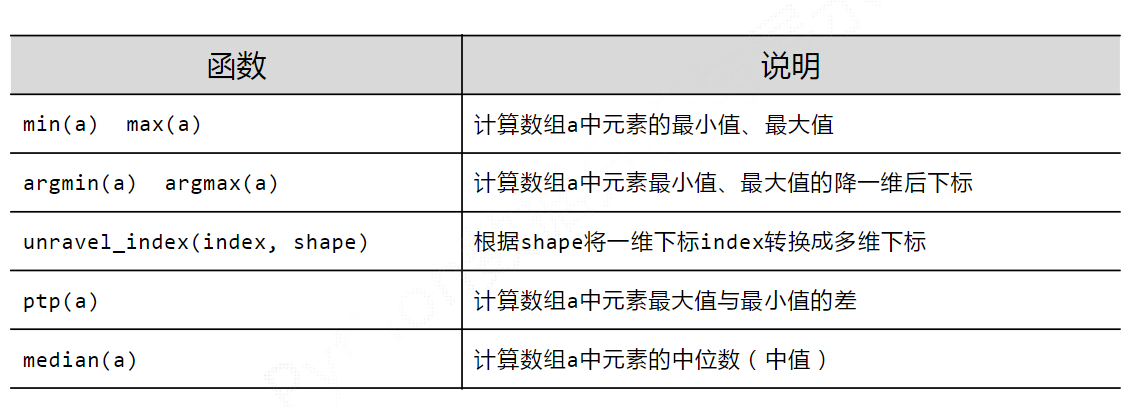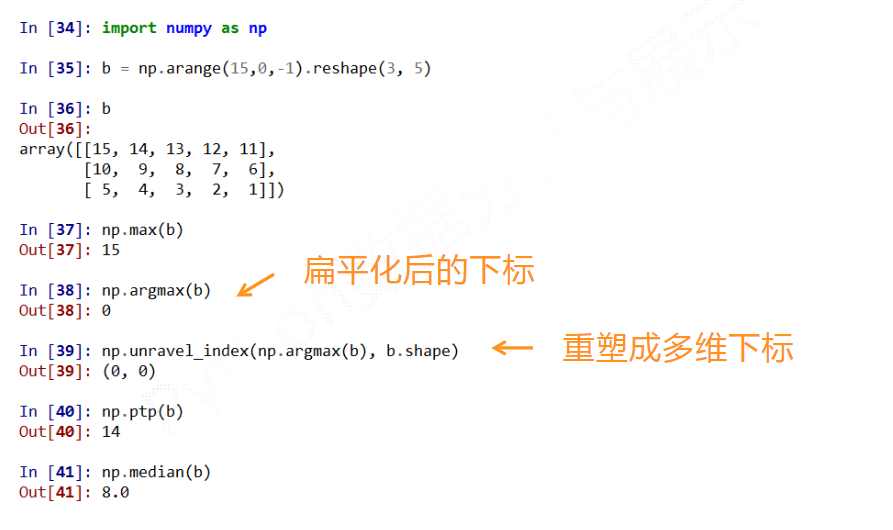## 梯度函数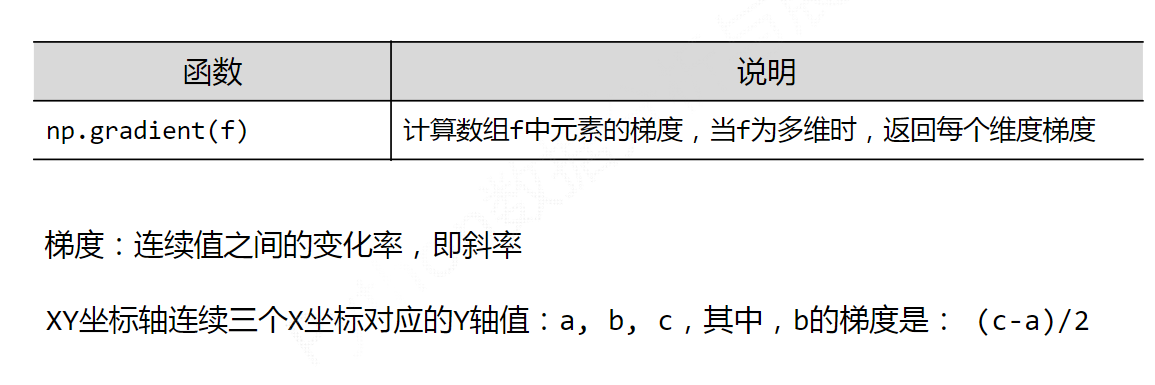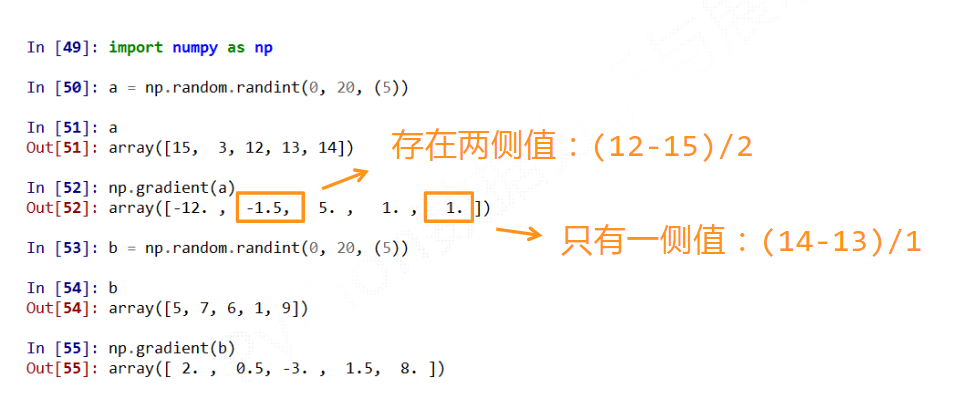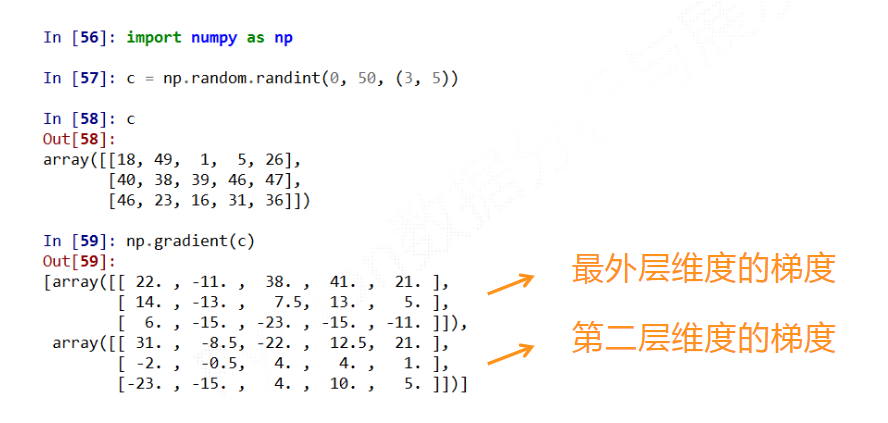## numpy线性代数

### 数组与标量之间的运算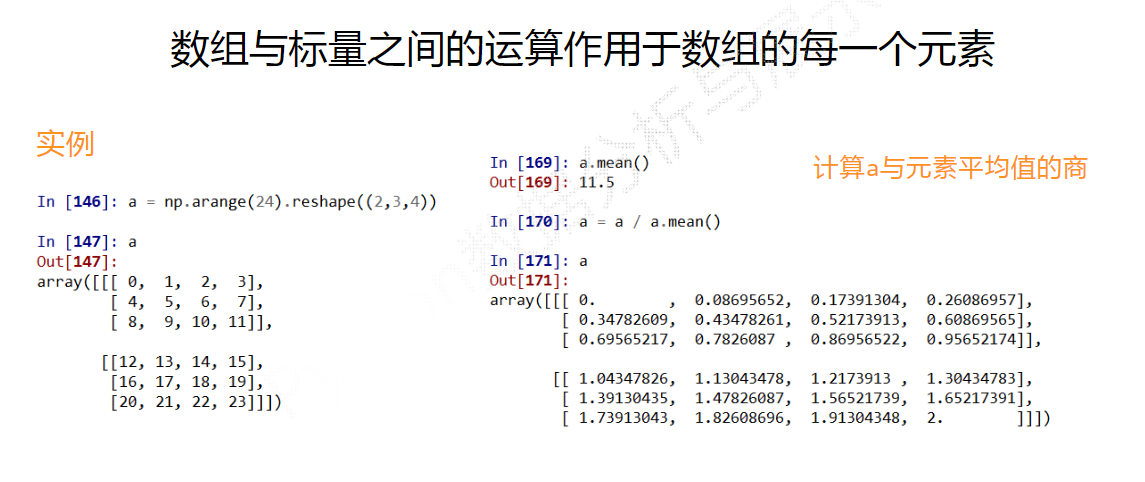### 常用numpy.linalg函数总结

函数功能
diag以一维数组的形式返回方阵的对角线元素，或将一维数组转换维方阵
dot矩阵乘法
trace计算对角线元素的和
det计算矩阵行列式
eig计算方阵的本征值和本征向量
inv计算方阵的逆
svd计算奇异值分解
solve解线性方程组Ax=b，其中A为方阵
lstsq计算Ax=b的最小二乘解

（好家伙，numpy内置函数太多了…）

展开全文python 机器学习
•python
• ## pythonnumpy中的复制（copy）

万次阅读 多人点赞 2019-08-31 22:53:23
使用python numpy中的数组复制 在使用python时我们经常会处理数组，有的时候是复制有的时候不是，这里也是初学者最容易误解的地方，简单讲，可以分为下面三种情况： 不是复制的情况（No Copy at All） import numpy ...python copy
• NumPy（Numerical Python的缩写）是一个开源的Python科学计算库。使用NumPy，就可以很自然地使用数组和矩阵。NumPy包含很多实用的数学函数，涵盖线性代数运算、傅里叶变换和随机数生成等功能。本文主要介绍一下NumPy...
• 计算Python Numpy向量之间的欧氏距离，已知vec1和vec2是两个Numpy向量，欧氏距离计算如下：import numpydist = numpy.sqrt(numpy.sum(numpy.square(vec1 - vec2)))或者直接：dist = numpy.linalg.norm(vec1 - vec2)...
• NumPy（Numerical Python的缩写）是一个开源的Python科学计算库。使用NumPy，就可以很自然地使用数组和矩阵。NumPy包含很多实用的数学函数，涵盖线性代数运算、傅里叶变换和随机数生成等功能。本文主要介绍一下NumPy...
• numpy中使用： 更改为任意维度：reshape() 展开为1维：flatten() 示例代码 import numpy as np if __name__ == '__main__': array = np.random.random(size=(5, 10)) # (5, 10) reshape_array = array.reshape...python
• image = np.expand_dims(a, axis=2) image = np.concatenate((image, image, image), axis=-1) 任务一：单通道图像转三通道理解/任务二：按axis=0或axis=1或axis=2（轴）堆叠（stack）python
• Python领域里有很多的与科学计算相关的包都或多或少地都使用到了Numpy这个模块包，例如著名的大数据包pandas就是基于numpy的，tensorflow的正常使用和安装也得首先安装numpy，由此可见学习numpy、学好numpy、深入...
• NumPy（Numerical Python的缩写）是一个开源的Python科学计算库。使用NumPy，就可以很自然地使用数组和矩阵。NumPy包含很多实用的数学函数，涵盖线性代数运算、傅里叶变换和随机数生成等功能。本文主要介绍一下NumPy...
• pythonnumpy.array函数 np.arry(object， dtype=None， *， copy=True， order='K'， subok=False，ndmin=0，array_like=None) 参数 object: 数组、公开数组接口的任何对象、其__array__方法返回数组的对象或任何...python
• 这是一个关于pythonnumpy科学计算包的帮助文档
• Python Numpy 排序，依据是指定行或者指定列排序
• numpy.mean()的使用 这个方法是用来计算给定数组沿指定轴的算术平均值的——即平均数 这是相关语法numpy.mean(arr, axis=None, dtype=float64) arr表示输入的数据，可以是一维数组或二维数组 axis表示计算平均数的轴...python
• 1、四舍五入方式在NumPy中主要有五种四舍五入方式：truncationfixroundingfloorceil2、trunc和fix删除小数，然后返回最接近零的浮点数。 使用trunc()和fix()函数。例如：截断以下数组的元素:import numpy as nparr =...
• Python numpy使用记录3.数组筛选切片，np.where前言np.where的使用1.筛选替换2.筛选提取 前言 如果我想提取数组中大于某个阈值的所有元素，可以使用数组筛选后提取。 本篇记录数组筛选的方法，np.where np.where的...python
• ## c++调用pythonnumpy编程

万次阅读 多人点赞 2019-10-10 11:33:57
找了很多的c++调用python的方法，首先可以肯定的有不止一种方式，直接使用python库、numpy arrayobject库来做；另外一种是使用boost/python boost/numpy的方式。后一种没有调通，是链接库的问...
• np.zeros_like(a)的目的是构建一个与a同维度的数组，并初始化所有变量为零。 def zeros_like(a, dtype=None, order='K', subok=True, shape=None): Return an array of zeros with the same shape and type as a ...python
• 计算矩阵R的行列式 ...import numpy as np # w1为列向量 x11 = np.array([[-3 / 4, -1 / 4, -1 / 8]]).T x12 = np.array([[5 / 4, -1 / 4, -1 / 8]]).T x13 = np.array([[5 / 4, -1 / 4, 7 / 8]]).Tpython
• numpy.std(arr, axis=None, dtype=float64) arr表示输入的数据，可以是一维数组或二维数组。 axis表示需要计算标准差的轴。 axis=0 表示沿列计算标准差， axis=1 表示沿行计算标准差 dtype表示计算标准差时使用的...
• Python numpy 提取矩阵的某一行或某一列的实例下面小编就为大家分享一篇Python numpy 提取矩阵的某一行或某一列的实例，具有很好的参考价值，希望对大家有所帮助。一起跟随小编过来看看吧如下所示：import numpy as ...
• 使用numpy模块对numpy.ndarray表示的图像左右翻转，即沿y轴翻转，函数为: numpy.fliplr(img) 示例 import cv2 import numpy as np img = cv2.imread("img.bmp", 1) img_lr = np.fliplr(img)python
• Python numpy.abs和abs别再傻傻分不清了说在最前先看示例程序-abs()函数再看示例程序-numpy.abs()函数观察两个程序的结果分析解释拓展总结最后 说在最前 终于在今天深深明白了真不能随便使用numpy.abs和abs… 别看这...python
• ## pythonnumpy.where()函数的用法

万次阅读 多人点赞 2018-11-09 15:31:16
numpy.where(condition[,x,y]) 返回元素，可以是x或y，具体取决于条件(condition) 如果只给出条件，则返回condition.nonzero()。 对于不同的输入，where返回的值是不同的。 参数： ccondition：array_llike，...python
• NumPy Random模块为此提供了两种方法：shuffle()和permutation()。 2、乱序排列 随机打乱意味着就地更改元素的排列。 即在数组本身中。 例如： 随机打乱下列数组的元素: from numpy import random import ...python...